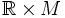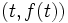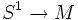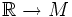Helix of a curve

Let$\gamma$ be a parametrized curve on a manifold$M$. The helix or spiral of$\gamma$ is a parametrized curve in$\R \times M$ that sends$t$ in the parameter domain to$(t,f(t))$. In other words, the helix of a curve is the Cartesian product of the curve with the identity-parametrized curve.
For a closed curve, viz a map$S^1 \to M$, we construct the helix using the map$\R \to M$ that composes the modulo map with the curve parametrization.